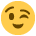#### Howdy, Stranger!

It looks like you're new here. If you want to get involved, click one of these buttons!

Supported by

# Bayes factor for equality of correlation coefficients?

edited November 2015

Hi,

I’ve recently collected data from two experiments in which I am interested in the correlations between various measures. In some cases I expect there to be a correlation but in other cases I am actually expecting no correlation.

The R code in Wetzels & Wagenmakers (2012)'s paper "A default Bayesian hypothesis test for correlations and partial correlations” has been very helpful in expressing the likelihood that a coefficient does not differ from 0.

What I would like to show now is that the coefficients from the second experiment are “the same” as the ones from the first experiment. That is, I’d like to compare a model that assumes that both are identical with a model that allows two parameters and would want to know the relative evidence the data provide for those two models. So basically the Bayesian version of this test: http://vassarstats.net/rdiff.html

I have found this blog post that seems to provide a Bayesian alternative to R's `cor.test()` function. It has JAGS code and I feel like there should be a way to do what I want to do in JAGS. So I'd look into that if there's no other way. I just wanted to ask here whether there's a more straightforward implementation of this somewhere that I can use?

Any pointers would be appreciated.Thanks!

• Florian

• edited 6:17AM

Hi Florian,

Note that JASP has a slightly different implementation of the correlation test than the one described in Wetzels et al. Specifically, JASP uses the original test as proposed by Jeffreys (for an explanation see http://www.ejwagenmakers.com/inpress/JeffreysToPTests.pdf).
We have not yet implemented a test between two correlations, but it is in the works. One way is to assume independent priors on both rate parameters; the other way is to assume a prior on the difference (and do a Savage-Dickey test on that difference). This would be facilitated by first transforming the correlation, perhaps using the Fisher z. The trick here is to define a good default prior. But, like I said, we have not done this yet and JASP does not do it.

Cheers,
E.J.

• edited 6:17AM

Hi EJ,

So, could I Fisher-z-transform both correlation coefficients, then subtract them and transform the difference back. Then test whether the difference is different from 0 using either JASP or the R-code from Ruud and your 2012 paper?

Or would that end up testing something different?

• edited 6:17AM

Hi Florian,
I don't think it's that simple, unfortunately. Once you have a posterior distribution for the difference in correlation coefficients, you need to "Savage-Dickey" this against a prior on the difference. Note that the scale on the difference between two correlation coefficients is not from -1 to 1, so you can't test it as you would a regular correlation. Of course if you have correlations for every participant individually (based on some repeated measures) then you can use ANOVA (but this is an unlikely scenario).
Cheers,
E.J.

• edited January 2016

Hi EJ,

unfortunately, this is not a repeated-measures situation.

Thank you for your quick and clear answers! I guess I'll have to wait until JASP includes this feature then.Take care!

• Florian
• edited 6:17AM

Hi EJ,

I want to quickly revive this thread to ask you another question. I recently asked the same question to someone else and they responded with this:

You can compute Bayes factors, as described in the paper, by comparing any two models.
Lets define two different models:

``````M_x: Z = b_xz X
M_y: Z = b_yz Y
``````

Similar to the linked paper, we can reframe the correlation test as a linear regression test. Particularly since in your case we only need to compare two vectors at a time so this is equivalent to testing that `b_xz != b_yz`. So

``````BF = p(Z | M_x) / p(Z | M_y)
``````

And then we have an identical setup to the partial correlation test (equation 15) except that the number of regression coefficients is fixed at 1.

``````# From Wetzels & Wagenmakers (2012):
corcor.bf=function(r1,r2,n){
int=function(r,n,g){
(1+g)^((n-2)/2)*(1+(1-r^2)*g)^(-(n-1)/2)*
g^(-3/2)*exp(-n/(2*g))};

bf10= integrate(int,lower=0,upper=Inf,r=r1,n=n)\$value/
integrate(int,lower=0,upper=Inf,r=r2,n=n)\$value
return(bf10)
}
``````

so...

``````set.seed(10010)
n <- 80
a <- rnorm(n)
b <- rnorm(n)
c <- a + rnorm(n, sd=2)

corcor.bf(cor(a,c), cor(a,b), 80)
BF ~= 86
``````

Is this a valid approach? I understand the conceptual part of the response and it makes sense to me but am not qualified to judge the modification of your (and Ruud's) function.

• Florian
• edited 6:17AM

I have not thought this through very deeply but I don't think it will work. Specifically, you want to know whether a correlation r1 (for experiment 1) is the same as r2 (for experiment 2). But experiment 1 and 2 provide different data. You have to compare two accounts for the complete data set, one with a single r and one with two r's. This is also the case when you use the regression framework: a single beta for the two sets of data, or two beta's.
E.J.

• edited 6:17AM

Hi EJ,

once again, thank you for your straightforward response. I see the difference between your regression framework and the one outlined above and why the latter might be problematic. Damn, I thought I stumbled across a neat solution...Thanks!

• Florian
• I noticed that this post is from a few years ago...i was curious if there was anything within JASP to do this now

that link is for comparing correlation from the Fishers Z transformation...is there a similar Bayesian test?

• We're still working on it, and I am pinging the person responsible every time this comes up :-)

• I appreciate your prompt response. I look forward to what you guys have in store for the future.